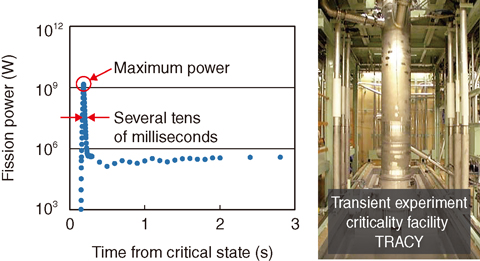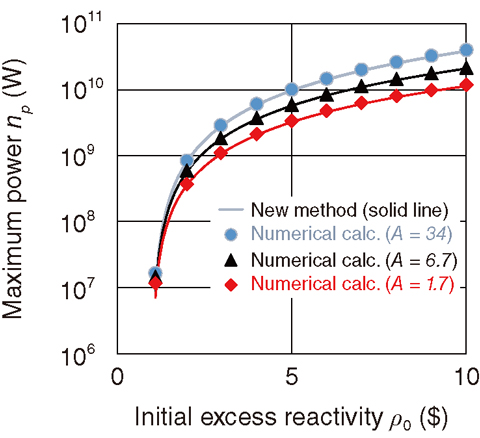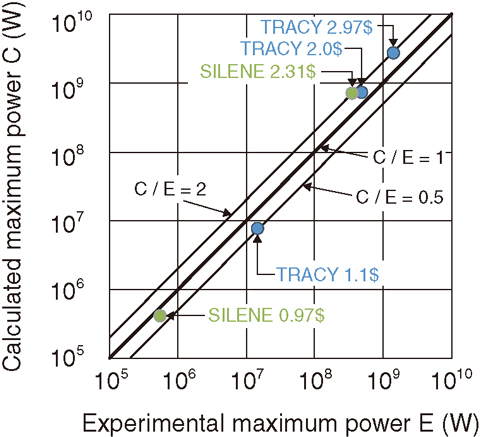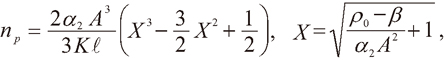# 2-4 Improvement of Severe Accident Assessment Method for Reprocessing Plants

## －Instant Calculation of the Maximum Power in Criticality Accidents－Fig.2-10　Data of a transient criticality experiment simulating a criticality accident using the transient experiment criticality facility (TRACY)

After a uranium solution is made critical by the instantaneous withdrawal of a neutron absorber at time zero, the power (i.e., the energy released from fissions per second) goes up and down in a very short period of time (about several ten ms), during which a lot of energy is released.Fig.2-11　Accuracy of the new method

The maximum power calculated using the new method agrees with the numerical calculation with the one-point kinetics code for a wide range of fuel compositions, indicated by the values of “A”.Fig.2-12　Comparison to experimental data

The maximum power calculated using the new method agrees with the experimental data. Low-enriched uranium (10%) was used in the TRACY experiment in Japan and high-enriched uranium (93%) was used in the SILENE experiment in France. “number \$” shows the initial excess reactivity.

The fuel reprocessing plant was designed to keep nuclear fuel subcritical. It is also important for confirming the safety of the public and workers in a postulated criticality accident. In a criticality accident, a lot of energy and radioactive rays are instantaneously released, as shown in Fig.2-10. The released energy mainly increases the temperature of the nuclear fuel and the radioactive ray causes the exposure of workers. The maximum value of the power, fission energy per second, provides information about the maximum strength of radioactive rays needed to assess the exposure of workers. The power profile is approximately expressed by the one-point kinetics equation; this equation is not easy to analytically solve because of its complexity. Alternatively, the equation is usually solved numerically using one-point kinetics codes. Such numerical calculations require a long time because the number of calculations depends on the number of cases, wherein there are many parameters such as the initial excess reactivity.

We focused on the fact that the fission power reaches its maximum value in a very short time, causing the one-point kinetics equation to be reduced to a simple form. We have analytically solved the simple equation to obtain the solution that expresses the maximum power as a function of parameters such as the initial reactivity, ρ0, reactivity temperature coefficients, α1, α2, reciprocal heat capacity, K, and delayed neutron fraction, β, as follows;where A = α1/(2α2). The maximum power calculated using this equation agrees with the numerical calculation, as shown in Fig.2-11. This result makes the calculation of the maximum power fast and easy. It also becomes easy to validate the calculation because the calculation procedure is clear. The comparison to experimental data shows good agreement for a wide range of maximum powers, as shown in Fig.2-12. The fast assessment of the dose of workers makes it possible to quickly employ countermeasures for safety.

As for the public dose, it is important to estimate the total amounts of fission products, for which the total fission energy must be estimated. We are planning to develop an expression for the released energy to make the assessment of the public dose much faster in the future.

| | | | |